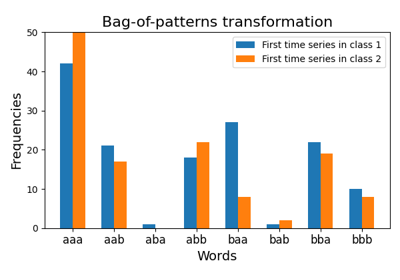# `pyts.transformation`.BagOfPatterns¶

class `pyts.transformation.``BagOfPatterns`(window_size=0.5, word_size=0.5, n_bins=4, strategy='normal', numerosity_reduction=True, window_step=1, norm_mean=True, norm_std=True, sparse=True, overlapping=True, alphabet=None)[source]

Bag-of-patterns representation for time series.

This algorithm uses a sliding window to extract subsequences from the time series and transforms each subsequence into a word using the Piecewise Aggregate Approximation and the Symbolic Aggregate approXimation algorithms. Thus it transforms each time series into a bag of words. Then it derives the frequencies of each word for each time series.

Parameters: window_size : int or float (default = 0.5) Length of the sliding window. If float, it represents a percentage of the size of each time series and must be between 0 and 1. word_size : int or float (default = 0.5) Length of the words. If float, it represents a percentage of the length of the sliding window and must be between 0. and 1. n_bins : int (default = 4) The number of bins to produce. It must be between 2 and `min(window_size, 26)`. strategy : ‘uniform’, ‘quantile’ or ‘normal’ (default = ‘normal’) Strategy used to define the widths of the bins: ‘uniform’: All bins in each sample have identical widths ‘quantile’: All bins in each sample have the same number of points ‘normal’: Bin edges are quantiles from a standard normal distribution numerosity_reduction : bool (default = True) If True, delete sample-wise all but one occurence of back to back identical occurences of the same words. window_step : int or float (default = 1) Step of the sliding window. If float, it represents the percentage of the size of each time series and must be between 0 and 1. The step of sliding window will be computed as `ceil(window_step * n_timestamps)`. norm_mean : bool (default = True) If True, center each subseries before scaling. norm_std : bool (default = True) If True, scale each subseries to unit variance. sparse : bool (default = True) Return a sparse matrix if True, else return an array. overlapping : bool (default = True) If True, time points may belong to two bins when decreasing the size of the subsequence with the Piecewise Aggregate Approximation algorithm. If False, each time point belong to one single bin, but the size of the bins may vary. alphabet : None or array-like, shape = (n_bins,) Alphabet to use. If None, the first n_bins letters of the Latin alphabet are used.

References

  J. Lin, R. Khade and Y. Li, “Rotation-invariant similarity in time series using bag-of-patterns representation”. Journal of Intelligent Information Systems, 39 (2), 287-315 (2012).

Examples

```>>> import numpy as np
>>> from pyts.transformation import BagOfPatterns
>>> X = np.arange(12).reshape(2, 6)
>>> bop = BagOfPatterns(window_size=4, word_size=4, sparse=False)
>>> bop.fit_transform(X)
array(...)
>>> bop.set_params(numerosity_reduction=False)
BagOfPatterns(...)
>>> bop.fit_transform(X)
array(...)
```
Attributes: vocabulary_ : dict A mapping of feature indices to terms.

Methods

 `__init__`([window_size, word_size, n_bins, …]) Initialize self. `fit`(X[, y]) Learn the dictionary. `fit_transform`(X[, y]) Derive word frequencies for each time series. `get_params`([deep]) Get parameters for this estimator. `set_params`(**params) Set the parameters of this estimator. `transform`(X) Derive word frequencies for each time series.
`__init__`(window_size=0.5, word_size=0.5, n_bins=4, strategy='normal', numerosity_reduction=True, window_step=1, norm_mean=True, norm_std=True, sparse=True, overlapping=True, alphabet=None)[source]

Initialize self. See help(type(self)) for accurate signature.

`fit`(X, y=None)[source]

Learn the dictionary.

Parameters: X : array-like, shape = (n_samples, n_timestamps) Input data y Ignored self : object
`fit_transform`(X, y=None)[source]

Derive word frequencies for each time series.

Parameters: X : array-like, shape = (n_samples, n_timestamps) Data to transform. y Ignored X_new : array, shape = (n_samples, n_words) Word frequencies.
`get_params`(deep=True)

Get parameters for this estimator.

Parameters: deep : bool, default=True If True, will return the parameters for this estimator and contained subobjects that are estimators. params : dict Parameter names mapped to their values.
`set_params`(**params)

Set the parameters of this estimator.

The method works on simple estimators as well as on nested objects (such as `Pipeline`). The latter have parameters of the form `<component>__<parameter>` so that it’s possible to update each component of a nested object.

Parameters: **params : dict Estimator parameters. self : estimator instance Estimator instance.
`transform`(X)[source]

Derive word frequencies for each time series.

Parameters: X : array-like, shape = (n_samples, n_timestamps) Data to transform. X_new : array, shape = (n_samples, n_words) Word frequencies.

## Examples using `pyts.transformation.BagOfPatterns`¶Bag of Patterns

Bag of Patterns